## Uniform flow across a mountain

One of the basic effects of topography is that when fluid crosses a topography relative vorticity values change since the potential vorticity is conserved (assuming that the fluid is invisvid).

This implies that a topography in a flow without relative vorticity creates relative vorticity, but only if there is also a background rotation, as can be seen from conservation of potential vorticity mentioned on the page about the basic effects of topography: f must be unequal zero.

Take for example the following example.

### Simulation

A circular mountain with a half cosine profile, a maximum height of 0.4 and a radius of 1 is placed in a square 6 by 6 domain. A uniform flow in the positive x-direction of V=(1.67,0) is applied. The constant part of the Coriolis parameter is f0=+3.

Immediately after the start of the simulation fluid going uphill aquires negative relative vorticity and fluid going downhill aquires positive relative vorticity: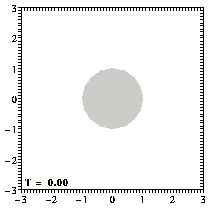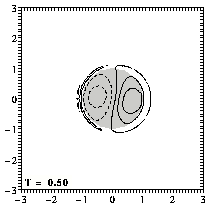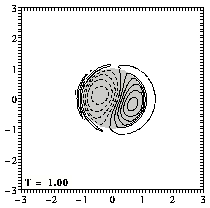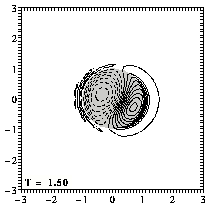Contours of relative vorticity: positive contours (0.1 to 2.0 at an interval of 0.1) are drawn solid, negative contours (-0.1 to -2.0 at an interval of -0.1) are drawn dashed, and the zero contour is dotted -- initially there is no relative vorticity in the domain. The mountain is indicated by the shaded circle.
(You can click on the pictures for a larger version.)

===> MPEG movie (288 kb; 51 frames) of the evolution until T=5

The maximum of vorticity grows in value as it climbs down the mountain. It reaches a maximum value when it has just reached the foot of the mountain. After that it remains constant (except for a small decay due to viscosity). The minimum of vorticity also increases in strength until about the same time.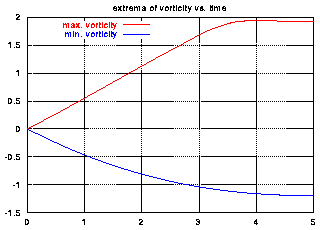Maximum (red) and minimum (blue) of relative
vorticity of the flow as function of time.

A tracer initially placed at the centre of the domain, i.e. at the centre of the mountain, moves thus: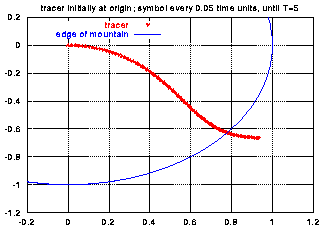Trajectory of a passive tracer (red symbols)
initially placed at the origin, that is: at the
centre of the mountain; the edge of the
mountain is shown with a blue line.

Clear from these pictures is that the flow is not symmetrical with respect to the x-axis, even though the topography and the uniform background flow and the rotation are. The reason is that the flow at the mountain is not symmetrical as soon as relative vorticity is created. This can be seen clearly when contours of the streamfunction are drawn:

### Some further remarks

• If there is a vortex in the domain, then this vortex can be responsible for the generation of the flow across the mountain, in stead of the uniform background flow used here.
See for example the simulations of a Bessel monopole encounters a circular mountain.
• If f0 is -3 rather than +3, the effects are opposite: positive relative vorticity is created at the mountain and negative behind is. The tracer's path is mirrored in the x-axis, and the vale of the maximum and minimum of vorticity are mirrored as well.
• If there is also a beta-effect in the rotation term, then the resulting flow is more complicated than shown above, because Rossby waves are generated (as in the example of a Bessel monopole on a beta-plane).
See for example Verron and Le Provost "A numerical study of quasi-geostrophic flow over isolated topography," J. Fluid Mech. 154, 231--252, 1985 -- who also apply a friction term, absent from these simulations.

The evolution of the vorticity distribution is computed with a Finite Difference Method which solves the two-dimensional vorticity (Navier-Stokes) equation. Time and distances are given in dimensionless units.

===> Some details on the computation presented on this page for those who are interested.